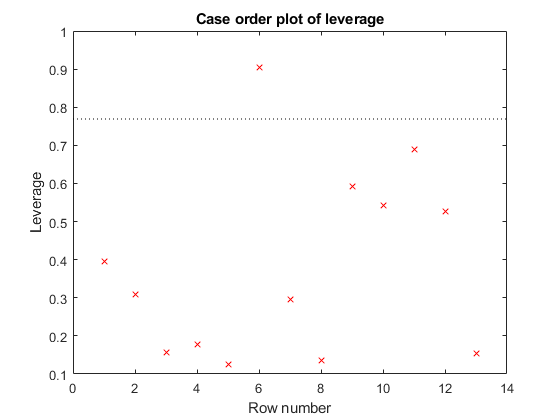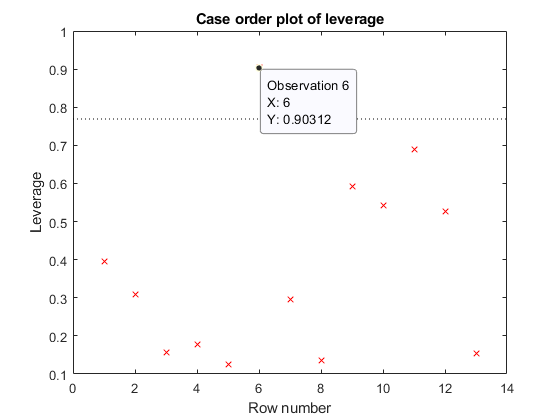# plotDiagnostics

Plot diagnostics of nonlinear regression model

## Syntax

``plotDiagnostics(mdl)``
``plotDiagnostics(mdl,plottype)``
``plotDiagnostics(mdl,plottype,Name,Value)``
``h = plotDiagnostics(___)``

## Description

`plotDiagnostics` creates a plot of observation diagnostics such as leverage or Cook's distance statistics to identify outliers and influential observations.

example

````plotDiagnostics(mdl)` creates a leverage plot of the nonlinear regression model (`mdl`) observations. A dotted line in the plot represents the recommended threshold values.```
````plotDiagnostics(mdl,plottype)` specifies the type of observation diagnostics `plottype`.```
````plotDiagnostics(mdl,plottype,Name,Value)` specifies the graphical properties of diagnostic data points using one or more name-value arguments. For example, you can specify the marker symbol and size for the data points.```
````h = plotDiagnostics(___)` returns graphics objects for the lines or contour in the plot using any of the input argument combinations in the previous syntaxes. Use `h` to modify the properties of a specific line or contour after you create the plot. For a list of properties, see Line Properties and Contour Properties.```

## Examples

collapse all

Create a leverage plot of a fitted nonlinear model, and find the points with high leverage.

Load the reaction data and fit a model of the reaction rate as a function of reactants.

```load reaction mdl = fitnlm(reactants,rate,@hougen,[1 .05 .02 .1 2]);```

Create a leverage plot of the fitted model.

`plotDiagnostics(mdl)`Use data tips to examine the observation with high leverage. A data tip appears when you hover over a data point.Alternatively, find the high-leverage observation at the command line.

`find(mdl.Diagnostics.Leverage > 0.8)`
```ans = 6```

## Input Arguments

collapse all

Nonlinear regression model object, specified as a `NonLinearModel` object created by using `fitnlm`.

Type of plot, specified as one of the values in this table.

ValuePlot TypeDotted Reference Line in Plot Purpose
`'contour'`Residual vs. leverage with overlaid contours of Cook's distanceContours of Cook's distanceIdentify observations with large residual values, high leverage, and large Cook's distance values.
`'cookd'`Cook's distanceRecommended threshold, computed by `3*mean(mdl.Diagnostics.CooksDistance)`Identify observations with large Cook's distance values.
`'leverage'`Leverage (Diagonal of Hat matrix)Recommended threshold, computed by `2*p/n`Identify high leverage observations.

For all plot types except `'contour'`, the x-axis is the row number (case order) of observations.

The `Diagnostics` property of `mdl` contains the diagnostic values used by `plotDiagnostics` to create plots.

### Name-Value Arguments

Specify optional pairs of arguments as `Name1=Value1,...,NameN=ValueN`, where `Name` is the argument name and `Value` is the corresponding value. Name-value arguments must appear after other arguments, but the order of the pairs does not matter.

Before R2021a, use commas to separate each name and value, and enclose `Name` in quotes.

Example: `'Color','blue','Marker','o'`

Note

The graphical properties listed here are only a subset. For a complete list, see Line Properties. The specified properties determine the appearance of diagnostic data points.

Line color, specified as the comma-separated pair consisting of `'Color'` and an RGB triplet, hexadecimal color code, color name, or short name for one of the color options listed in the following table.

The `'Color'` name-value pair argument also determines marker outline color and marker fill color if `'MarkerEdgeColor'` is `'auto'` (default) and `'MarkerFaceColor'` is `'auto'`.

For a custom color, specify an RGB triplet or a hexadecimal color code.

• An RGB triplet is a three-element row vector whose elements specify the intensities of the red, green, and blue components of the color. The intensities must be in the range `[0,1]`, for example, ```[0.4 0.6 0.7]```.

• A hexadecimal color code is a string scalar or character vector that starts with a hash symbol (`#`) followed by three or six hexadecimal digits, which can range from `0` to `F`. The values are not case sensitive. Therefore, the color codes `"#FF8800"`, `"#ff8800"`, `"#F80"`, and `"#f80"` are equivalent.

Alternatively, you can specify some common colors by name. This table lists the named color options, the equivalent RGB triplets, and hexadecimal color codes.

Color NameShort NameRGB TripletHexadecimal Color CodeAppearance
`"red"``"r"``[1 0 0]``"#FF0000"``"green"``"g"``[0 1 0]``"#00FF00"``"blue"``"b"``[0 0 1]``"#0000FF"``"cyan"` `"c"``[0 1 1]``"#00FFFF"``"magenta"``"m"``[1 0 1]``"#FF00FF"``"yellow"``"y"``[1 1 0]``"#FFFF00"``"black"``"k"``[0 0 0]``"#000000"``"white"``"w"``[1 1 1]``"#FFFFFF"``"none"`Not applicableNot applicableNot applicableNo color

Here are the RGB triplets and hexadecimal color codes for the default colors MATLAB® uses in many types of plots.

`[0 0.4470 0.7410]``"#0072BD"``[0.8500 0.3250 0.0980]``"#D95319"``[0.9290 0.6940 0.1250]``"#EDB120"``[0.4940 0.1840 0.5560]``"#7E2F8E"``[0.4660 0.6740 0.1880]``"#77AC30"``[0.3010 0.7450 0.9330]``"#4DBEEE"``[0.6350 0.0780 0.1840]``"#A2142F"`Example: `'Color','blue'`

Line width, specified as the comma-separated pair consisting of `'LineWidth'` and a positive value in points. If the line has markers, then the line width also affects the marker edges.

Example: `'LineWidth',0.75`

Marker symbol, specified as the comma-separated pair consisting of `'Marker'` and one of the values in this table.

MarkerDescriptionResulting Marker
`"o"`Circle`"+"`Plus sign`"*"`Asterisk`"."`Point`"x"`Cross`"_"`Horizontal line`"|"`Vertical line`"square"`Square`"diamond"`Diamond`"^"`Upward-pointing triangle`"v"`Downward-pointing triangle`">"`Right-pointing triangle`"<"`Left-pointing triangle`"pentagram"`Pentagram`"hexagram"`Hexagram`"none"`No markersNot applicable

Example: `'Marker','+'`

Marker outline color, specified as the comma-separated pair consisting of `'MarkerEdgeColor'` and an RGB triplet, hexadecimal color code, color name, or short name for one of the color options listed in the `Color` name-value pair argument.

The default value of `'auto'` uses the same color specified by using `'Color'`.

Example: `'MarkerEdgeColor','blue'`

Marker fill color, specified as the comma-separated pair consisting of `'MarkerFaceColor'` and an RGB triplet, hexadecimal color code, color name, or short name for one of the color options listed in the `Color` name-value pair argument.

The `'auto'` value uses the same color specified by using `'Color'`.

Example: `'MarkerFaceColor','blue'`

Marker size, specified as the comma-separated pair consisting of `'MarkerSize'` and a positive value in points.

Example: `'MarkerSize',2`

## Output Arguments

collapse all

Graphics objects corresponding to the lines or contour in the plot, returned as a graphics array. Use dot notation to query and set properties of the graphics objects. For details, see Line Properties and Contour Properties.

You can use name-value arguments to specify the appearance of diagnostic data points corresponding to the first graphics object `h(1)`.

collapse all

### Hat Matrix

The hat matrix H is defined in terms of the data matrix X and the Jacobian matrix J:

`${J}_{i,j}={\frac{\partial f}{\partial {\beta }_{j}}|}_{{x}_{i},\beta }$`

Here f is the nonlinear model function, and β is the vector of model coefficients.

The Hat Matrix H is

H = J(JTJ)–1JT.

The diagonal elements Hii satisfy

`$\begin{array}{l}0\le {h}_{ii}\le 1\\ \sum _{i=1}^{n}{h}_{ii}=p,\end{array}$`

where n is the number of observations (rows of X), and p is the number of coefficients in the regression model.

### Leverage

Leverage is a measure of the effect of a particular observation on the regression predictions due to the position of that observation in the space of the inputs.

The leverage of observation i is the value of the ith diagonal term hii of the hat matrix H. Because the sum of the leverage values is p (the number of coefficients in the regression model), an observation i can be considered an outlier if its leverage substantially exceeds p/n, where n is the number of observations.

### Cook’s Distance

The Cook’s distance Di of observation i is

`${D}_{i}=\frac{\sum _{j=1}^{n}{\left({\stackrel{^}{y}}_{j}-{\stackrel{^}{y}}_{j\left(i\right)}\right)}^{2}}{p\text{\hspace{0.17em}}MSE},$`

where

• ${\stackrel{^}{y}}_{j}$ is the jth fitted response value.

• ${\stackrel{^}{y}}_{j\left(i\right)}$ is the jth fitted response value, where the fit does not include observation i.

• MSE is the mean squared error.

• p is the number of coefficients in the regression model.

Cook’s distance is algebraically equivalent to the following expression:

`${D}_{i}=\frac{{r}_{i}^{2}}{p\text{\hspace{0.17em}}MSE}\left(\frac{{h}_{ii}}{{\left(1-{h}_{ii}\right)}^{2}}\right),$`

where ei is the ith residual.

## Tips

• The data cursor displays the values of the selected plot point in a data tip (small text box located next to the data point). The data tip includes the x-axis and y-axis values for the selected point, along with the observation name or number.

 Neter, J., M. H. Kutner, C. J. Nachtsheim, and W. Wasserman. Applied Linear Statistical Models, Fourth Edition. Irwin, Chicago, 1996.